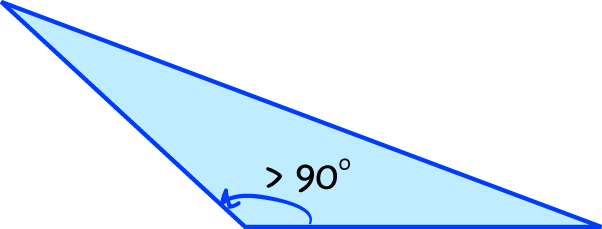# Definition of Obtuse TriangleAn obtuse triangle is a triangle in which one of the angles is bigger that $90^\circ$.

Of course, since the angle sum of a triangle is $180^\circ$, an obtuse triangle can only contain one obtuse angle.

### Description

The aim of this dictionary is to provide definitions to common mathematical terms. Students learn a new math skill every week at school, sometimes just before they start a new skill, if they want to look at what a specific term means, this is where this dictionary will become handy and a go-to guide for a student.

### Audience

Year 1 to Year 12 students

### Learning Objectives

Learn common math terms starting with letter O

Author: Subject Coach
You must be logged in as Student to ask a Question.